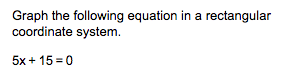# Graph the following equation in a rectangularcoordinate system5x 15 0

Question
1 viewshelp_outlineImage TranscriptioncloseGraph the following equation in a rectangular coordinate system 5x 15 0 fullscreen
check_circle

Step 1

Consider the given equation as 5x + 15=0.

Since the equation is line...

### Want to see the full answer?

See Solution

#### Want to see this answer and more?

Solutions are written by subject experts who are available 24/7. Questions are typically answered within 1 hour.*

See Solution
*Response times may vary by subject and question.
Tagged in

### Other